Related Articles
Block Cipher modes of Operation
• Last Updated : 09 Aug, 2019

Encryption algorithms are divided into two categories based on input type, as block cipher and stream cipher. Block cipher is an encryption algorithm which takes fixed size of input say b bits and produces a ciphertext of b bits again. If input is larger than b bits it can be divided further. For different applications and uses, there are several modes of operations for a block cipher.

Electronic Code Book (ECB) –
Electronic code book is the easiest block cipher mode of functioning. It is easier because of direct encryption of each block of input plaintext and output is in form of blocks of encrypted ciphertext. Generally, if a message is larger than b bits in size, it can be broken down into bunch of blocks and the procedure is repeated.

Procedure of ECB is illustrated below: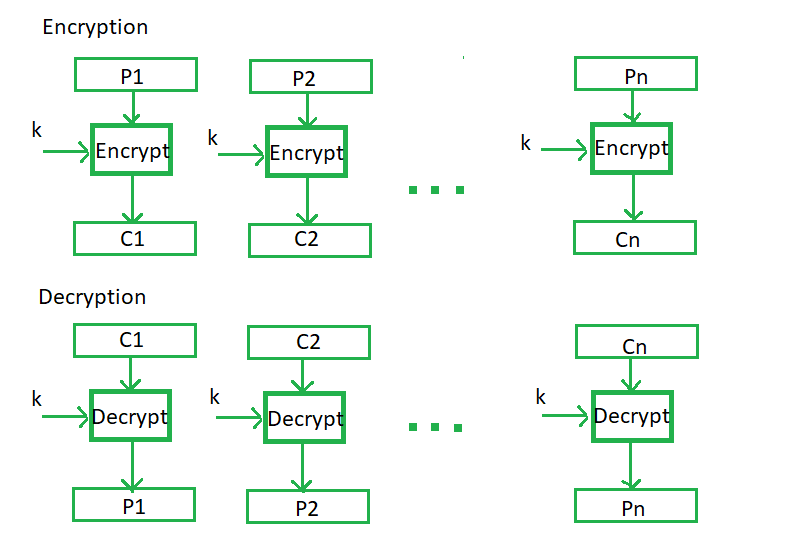Advantages of using ECB –

• Parallel encryption of blocks of bits is possible, thus it is a faster way of encryption.
• Simple way of block cipher.

Disadvantages of using ECB –

• Prone to cryptanalysis since there is a direct relationship between plaintext and ciphertext.

Cipher Block Chaining –
Cipher block chaining or CBC is an advancement made on ECB since ECB compromises some security requirements. In CBC, previous cipher block is given as input to next encryption algorithm after XOR with original plaintext block. In a nutshell here, a cipher block is produced by encrypting a XOR output of previous cipher block and present plaintext block.

The process is illustrated here: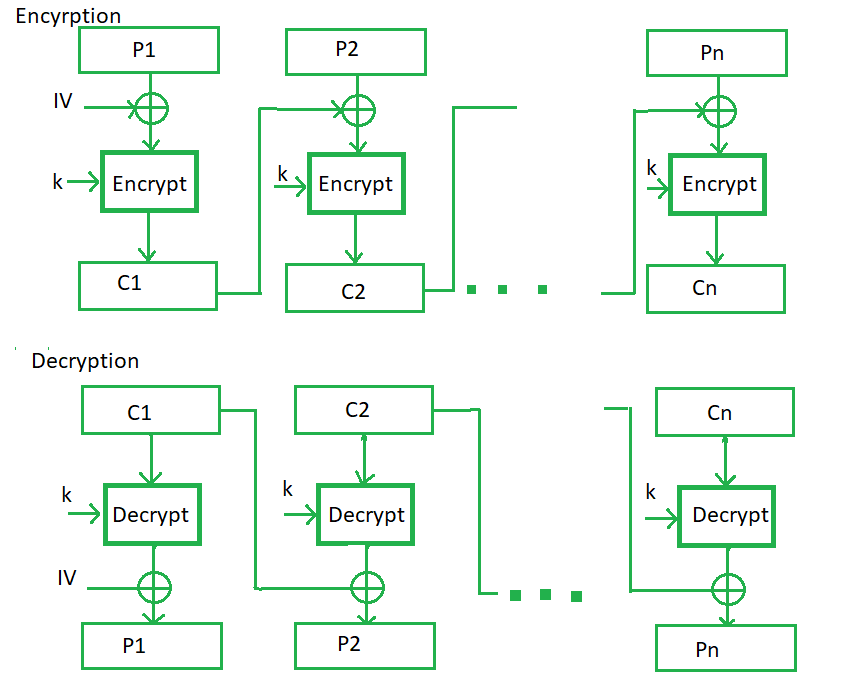Advantages of CBC –

• CBC works well for input greater than b bits.
• CBC is a good authentication mechanism.
• Better resistive nature towards cryptanalsis than ECB.

Disadvantages of CBC –

• Parallel encryption is not possible since every encryption requires previous cipher.

Cipher Feedback Mode (CFB) –
In this mode the cipher is given as feedback to the next block of encryption with some new specifications: first an initial vector IV is used for first encryption and output bits are divided as set of sandb-s bits the left hand side sbits are selected and are applied an XOR operation with plaintext bits. The result given as input to a shift register and the process continues. The encryption and decryption process for the same is shown below, both of them use encryption algorithm.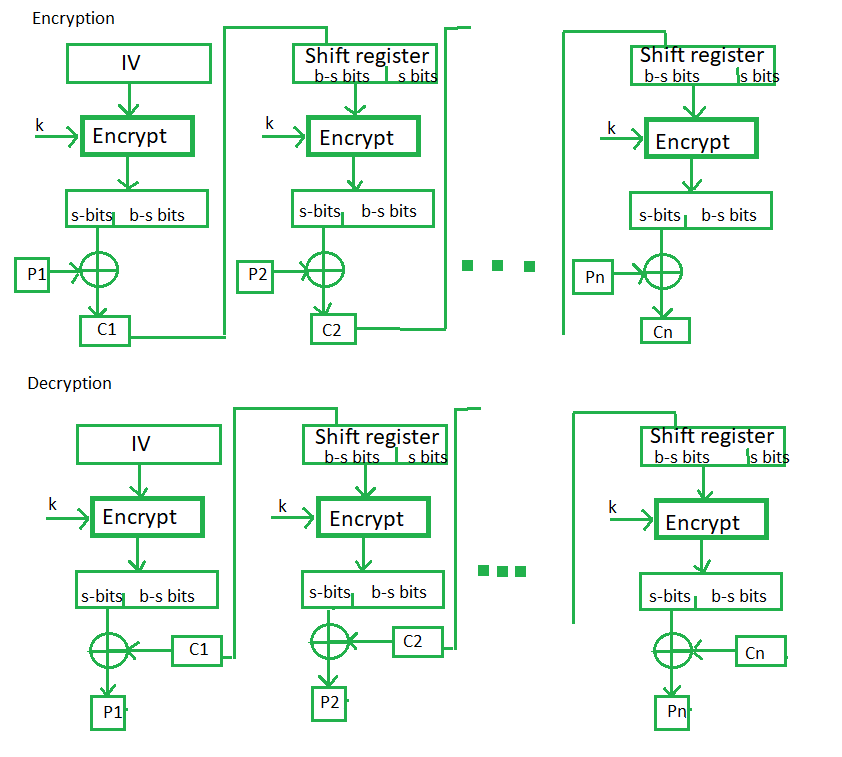Advantages of CFB –

• Since, there is some data loss due to use of shift register, thus it is difficult for applying cryptanalysis.

Output Feedback Mode –
The output feedback mode follows nearly same process as the Cipher Feedback mode except that it sends the encrypted output as feedback instead of the actual cipher which is XOR output. In this output feedback mode, all bits of the block are send instead of sending selected s bits. The Output Feedback mode of block cipher holds great resistance towards bit transmission errors. It also decreases dependency or relationship of cipher on plaintext.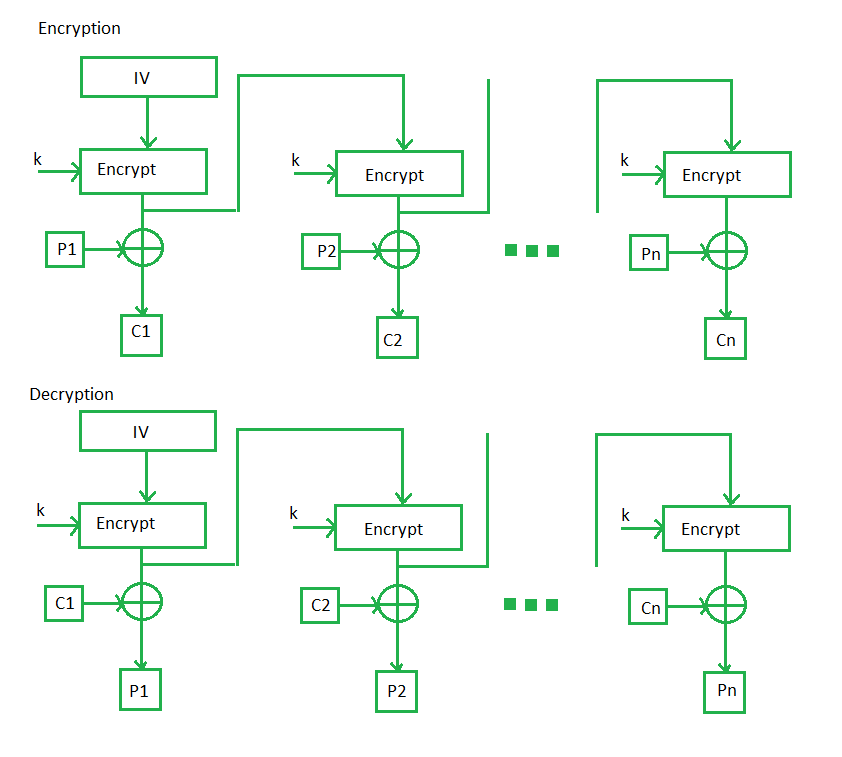Counter Mode –
The Counter Mode or CTR is a simple counter based block cipher implementation. Every time a counter initiated value is encrypted and given as input to XOR with plaintext which results in ciphertext block. The CTR mode is independent of feedback use and thus can be implemented in parallel.

Its simple implementation is shown below: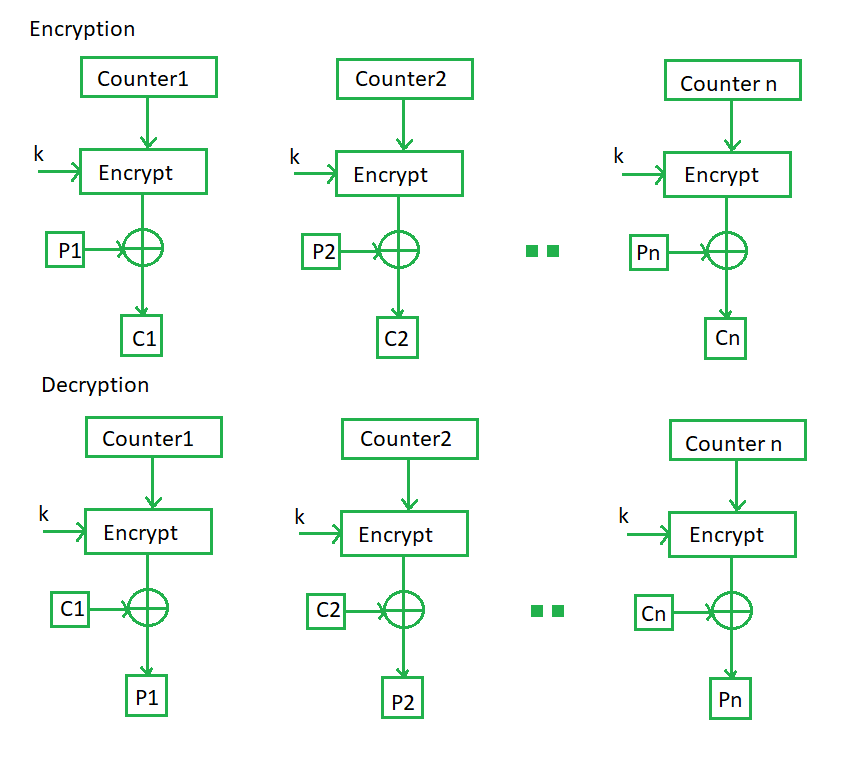Attention reader! Don’t stop learning now. Get hold of all the important CS Theory concepts for SDE interviews with the CS Theory Course at a student-friendly price and become industry ready.

My Personal Notes arrow_drop_up